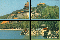#Q-Puzzle

This is a mathematical jigsaw puzzle! You have a photo cut into $q×q$ pieces, where $q$ is a power of a prime $p$. Therefore it represents the vector plane over the finite field ${𝔽}_{q}$ with $q$ elements.

These $q×q$ pieces will be presented to you in disorder, result of a (hidden) affine transformation of the plane. And your goal is to recover the image by finding the inverse affine transformation. You have the right to multiple tries in order to do it.

Recall that an affine transformation is a linear transformation followed by a translation. All are operations over ${𝔽}_{q}$.

You may play this game even without understanding the mathematics behind. In this case we suggest you don't go further than than $q=5$ otherwise the game risks to be very frustrating.

We also recommend another online jigsaw Shifting puzzle which requires much less mathematical background.
• Choose : = (Bigger usually means more difficult puzzle.)
• =
In order to access WIMS services, you need a browser supporting forms. In order to test the browser you are using, please type the word wims here: and press Enter''.